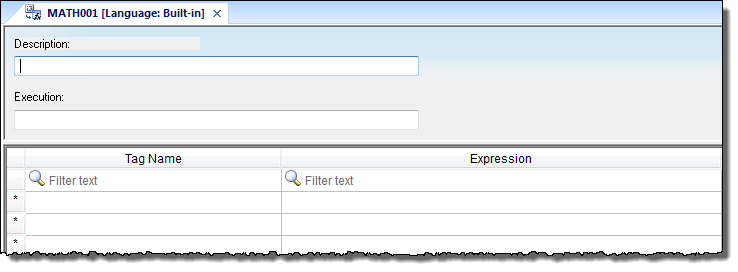# Math worksheet

A Math worksheet is used to implement program logic (using the Built-in Scripting Language) that should be continuously executed during runtime, rather than on specific actions like the user pressing a button on a screen.

Note: The Math worksheet is functionally similar to the Script worksheet, except that it uses the Built-in Scripting Language instead of VBScript.
To create a new Math worksheet, do one of the following:
• On the Insert tab of the ribbon, in the Task Worksheets group, click Math;
• Right-click the Math folder in the Project Explorer, and then click Insert on the shortcut menu; or
• Click New on the Application menu, click the File tab, and then select Math Worksheet.

To edit an existing Math worksheet, double-click it in the Project Explorer.

Figure 1. Math worksheetThe Math worksheet is divided into two areas:
• Header area (top section), which contains information for the whole group; and
• Body area (bottom section), where you define each tag, expression, and Programming Lines (logical routines and mathematical calculations through functions and logical operations) in the group.
Use the Header parameters on this worksheet as follows:
• Description field: Type a description of the worksheet for documentation purposes.
• Execution field: Type an expression, a single tag, or a constant value to determine when the worksheet should execute.
Note: The project executes the worksheet only when the Execution field result is not zero. If you always want the worksheet to execute, type a 1 (constant value) in the Execution field.
Use the Body parameters on this worksheet as follows:
• Tag Name field: Type a tag to receive a return value from the specified calculation in the Expression column.
• Expression field: Type an expression to return a return value to the specified tag in the Tag Name column.

To execute a Math worksheet at a specific time, separate from whatever is configured in the Execution field, use the Math function anywhere an expression is allowed.